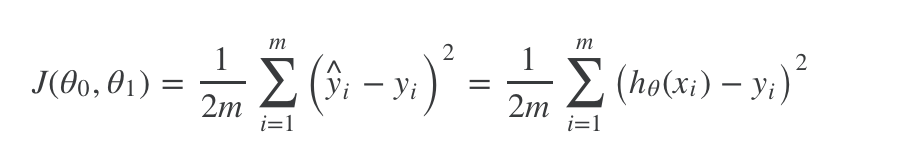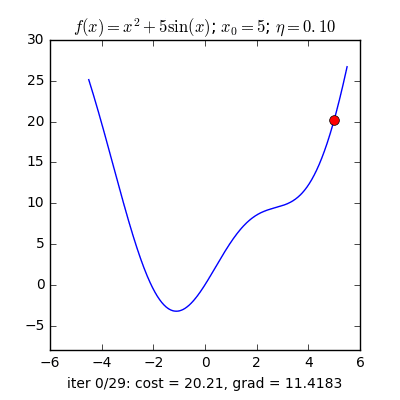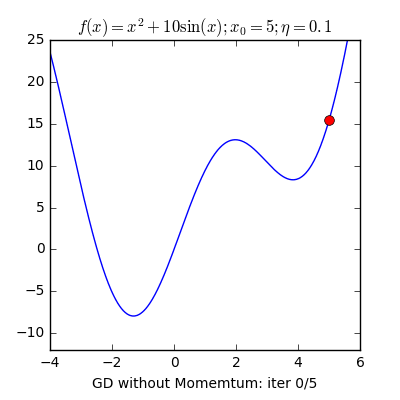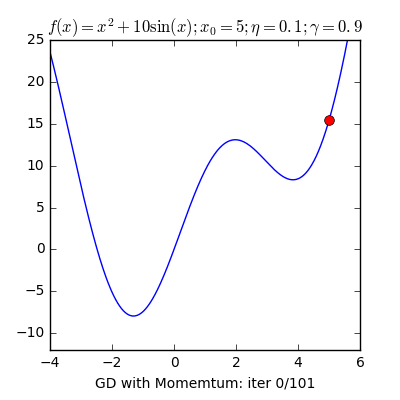### 1. What is gradient descent

Gradient Descent (GD) is an optimization algorithm used for minimizing the cost function in Machine Learning and Deep Learning.

A easy way to understand : Gradient Descent is one of algorithm to training your model in Machine Learning and Deep Learning.

Like I said , Gradient Decsent is algorithm used for minimizing cost function , so if we have cost function like this :and we can define Gradient Descent like :where j = 0 and j = 1

### 3. How Gradient Descent work

Firs , we initialization θj and assign θj into algorithm to compute new θj and repeat until find out θ so that cost function J(θj) is small enough.
Like this :### 4. Type of Gradient Descent